# Class 10 Maths Important Questions for Application of Trigonometry

Question 1.
A person, standing on the bank of a river, observes that the angle subtended by a tree on the opposite bank is 60°. When he retreated 20m from the bank, he finds the angle to be 30°. Find the height of the tree and the breadth of the river.

Question 2.
A tree is broken by the wind. The top struck the ground at an angle of 30° and at a distance of 30 meters from the roots. Find the whole height of the tree.

Question 3.
The angles of elevation of the top of a tower from two points at distances p and q meters from the base and in the same straight line with it are complementary. Prove that the height of the tower is $\sqrt {pq}$

Question 4.
At a point on level ground, the angle of elevation of a vertical tower is found to be such that its tangent is 5/12 . On walking 192 meters towards the tower, the tangent of the angle of elevation is 3/4. Find the height of the tower.

Question 5.
A person standing on the bank of a river observes that the angle of elevation of the top of a tree standing on the opposite bank is 60o. When he moves 40 meters away from the bank, he finds the angle of elevation to be 30°. Find the height of the tree and the width of the river.

Question 6.
A man on the top of a vertical tower observes a car moving at a uniform speed coming directly towards it. If it takes 12 minutes for the angle of depression to change from 30° to 45°, how soon after this, will the car reach the tower? Give your answer to the nearest second.

Question 7.
The shadow of a flag – staff is three times as long as the shadow of the flag – staff when the sun rays meet the ground at an angle of 60o. Find the angle between the sun rays and the ground at the time of longer shadow.

Question 8.
An aeroplane at an altitude of 200m observes the angles of depression of opposite points on the two banks of a river to be 45° and 60o. Find the width of the river.

Question 9.
Two pillars of equal height and on either side of a road, which is 100m wide. The angles of elevation of the top of the pillars are 60o and 30° at a point on the road between the pillars. Find the position of the point between the pillars and the height of each pillar.

Question 10.
The angle of elevation of a jet plane from a point A on the ground is 60°. After a flight of 30 seconds, the angle of elevation changes to 30o. If the jet plane is flying at a constant height of $3600 \sqrt {3}$m, find the speed of the jet plane.

Question 11.
If the angle of elevation of a cloud from a point h meters above a lake is $\alpha$ and the angle of depression of its reflection in the lake is $\beta$, prove that the height of the cloud is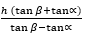Question 12.
A round balloon of radius r subtends an angle α at the eye of the observer while the angle of elevation of its centre is $\beta$. Prove that the height of the centre of the balloon is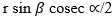Question 13.
The angle of elevation of a cliff from a fixed point is $\theta$. After going up a distance of K meters towards the top of the cliff at an angle of $\phi$, it is found that the angle of elevation is $\alpha$. Show that the height of the cliff is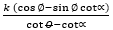meter

Question 14.
The angle of elevation of the top of a vertical tower PQ from a point X on the ground is 60°. At a point Y, 40m vertically above X, the angle of elevation of the top is 45° Calculate the height of the tower.

Question 15.
If the angle of elevation of a cloud from a point h metre above a lake is $\alpha$ and the angle of depression of its reflection in the lake be $\beta$, prove that the distance of the cloud from the point of observation is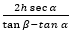Question 16.
From an aeroplane vertically above a straight horizontal road, the angles of depression of two consecutive mile stones on opposite sides of the aeroplane are observed to be $\alpha$ and $\beta$. Show that the height in miles of aeroplane above the road is given by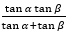Question 17.
A ladder rests against a wall at an angle $\alpha$ to the horizontal. Its foot is pulled away from the wall through a distance , so that its slides a distance b down the wall making an angle $\beta$ with the horizontal. Show that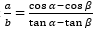Question 18.
A boy is standing on the ground and flying a kite with 100m of string at an elevation of 30°. Another boy is standing on the roof of a 10 m high building and is flying his kite at an elevation of 45°. Both the boys are on opposite sides of both the kites. Find the length of the string that the second boy must have so that the two kites meet.

Question 19.
Two boats approach a light house in mid- sea from opposite directions. The angles of elevation of the top of the light house from two boats are 30° and 45° respectively. If the distance between two boats is 100m, find the height of the light house.

Question 20.
From the top of a light house, the angles of depression of two ships on the opposite sides of it are observed to be $\alpha$ and $\beta$. If the height of the light house be h meters and the line joining the ships passes through the foot of the light house, show that the distance between the ship is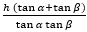metres

Question 21.
As observed from the top of a 150m tall light house, the angles of depression of two ships approaching it are 30° and °. If one ship is directly behind the other, find the distance between the two ships.

## Summary

This Class 10 Maths Important Questions for Application of Trigonometry with answers is prepared keeping in mind the latest syllabus of CBSE . This has been designed in a way to improve the academic performance of the students. If you find mistakes , please do provide the feedback on the mail.You can download in PDF form also using the below linksGo back to Class 10 Main Page using below links

### Practice Question

Question 1 What is $1 - \sqrt {3}$ ?
A) Non terminating repeating
B) Non terminating non repeating
C) Terminating
D) None of the above
Question 2 The volume of the largest right circular cone that can be cut out from a cube of edge 4.2 cm is?
A) 19.4 cm3
B) 12 cm3
C) 78.6 cm3
D) 58.2 cm3
Question 3 The sum of the first three terms of an AP is 33. If the product of the first and the third term exceeds the second term by 29, the AP is ?
A) 2 ,21,11
B) 1,10,19
C) -1 ,8,17
D) 2 ,11,20